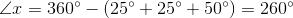Example Questions

2 Next →

Example Question #11 : How To Find An Angle In A Kite

A kite has one set of opposite interior angles where the two angles measure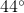and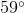, respectively. Find the measurement for one of the two remaining interior angles in this kite.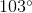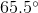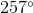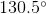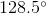Explanation:

The sum of the interior angles of any polygon can be found by applying the formula: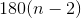degrees, whereis the number of sides in the polygon.

By definition, a kite is a polygon with four total sides (quadrilateral). The sum of the interior angles of any quadrilateral must equal: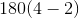degrees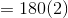degrees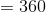degrees. Additionally, kites must have two sets of equivalent adjacent sides & one set of congruent opposite angles

The missing angle can be found by finding the sum of the non-congruent opposite angles. Then divide the difference between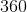degrees and the non-congruent opposite angles sum by: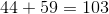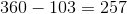This means that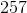is the sum of the remaining two angles, which must be opposite congruent angles. Therefore, the measurement for one of the angles is: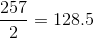Example Question #12 : How To Find An Angle In A Kite

A kite has one set of opposite interior angles where the two angles measure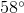and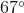, respectively. Find the measurement of the sum of the two remaining interior angles.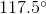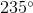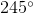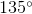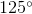Explanation:

The sum of the interior angles of any polygon can be found by applying the formula:degrees, whereis the number of sides in the polygon.

By definition, a kite is a polygon with four total sides (quadrilateral). The sum of the interior angles of any quadrilateral must equal:degreesdegreesdegrees. Additionally, kites must have two sets of equivalent adjacent sides & one set of congruent opposite angles

To find the sum of the remaining two angles, determine the difference betweendegrees and the sum of the non-congruent opposite angles.

The solution is: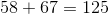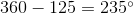This means thatis the sum of the remaining two opposite angles.

Example Question #13 : How To Find An Angle In A Kite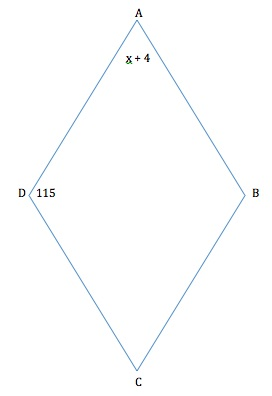If the diagonals of the quadrilateral above were drawn in the figure, they would form four 90 degree angles at the center. In degrees, what is the value of?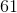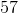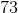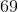Explanation:

A quadrilateral is considered a kite when one of the following is true:

(1) it has two disjoint pairs of sides are equal in length or

(2) one diagonal is the perpendicular bisector of the other diagonal. Given the information in the question, we know (2) is definitely true.

To findwe must first find the values of all of the angles.

The sum of angles within any quadrilateral is 360 degrees.

Therefore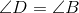.

To find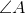: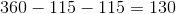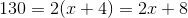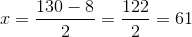Example Question #14 : How To Find An Angle In A Kite

In a particular kite, one angle that lies between congruent sides measures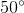, and one angle that lies between non-congruent sides measures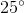. What is the measure of the angle opposite theangle?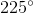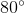One of the rules governing kites is that the angles which lie between non-congruent sides are congruent to each other. Thus, we know one of the missing angles is also. Since all angles in a quadrilateral must sum to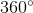, we know that the other missing angle is